### Specifying the State Space Model

Instead of allowing the STATESPACE procedure to select the model automatically, you can use FORM and RESTRICT statements to specify a state space model.

#### Specifying the State Vector

Use the FORM statement to control the form of the state vector. You can use this feature to force PROC STATESPACE to estimate and forecast a model different from the model it would select automatically. You can also use this feature to reestimate the automatically selected model (possibly with restrictions) without repeating the canonical correlation analysis.

The FORM statement specifies the number of lags of each variable to include in the state vector. For example, the statement FORM X 3; forces the state vector to include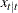,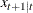, and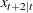. The following statement specifies the state vector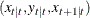, which is the same state vector selected in the preceding example:

```   form x 2 y 1;
```

You can specify the form for only some of the variables and allow PROC STATESPACE to select the form for the other variables. If only some of the variables are specified in the FORM statement, canonical correlation analysis is used to determine the number of lags included in the state vector for the remaining variables not specified by the FORM statement. If the FORM statement includes specifications for all the variables listed in the VAR statement, the state vector is completely defined and the canonical correlation analysis is not performed.

#### Restricting the F and G matrices

After you know the form of the state vector, you can use the RESTRICT statement to fix some parameters in the
andmatrices to specified values. One use of this feature is to remove insignificant parameters by restricting them to 0.

In the introductory example shown in the preceding section, the F[2,3] parameter is not significant. (The parameters estimation output shown in Figure 28.6 gives the t statistic for F[2,3] as –0.06. F[3,3] and F[3,1] also have low significance with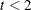.)

The following statements reestimate this model with F[2,3] restricted to 0. The FORM statement is used to specify the state vector and thus bypass the canonical correlation analysis.

```proc statespace data=in out=out lead=10;
var x(1) y(1);
id t;
form x 2 y 1;
restrict f(2,3)=0;
run;
```

The final estimates produced by these statements are shown in Figure 28.10.

Figure 28.9: Results Using RESTRICT Statement

The STATESPACE Procedure
Selected Statespace Form and Fitted Model

State Vector
x(T;T) y(T;T) x(T+1;T)

Estimate of Transition Matrix
0 0 1
0.290051 0.467468 0
0.227051 0.226139 0.26436

Input Matrix for Innovation
1 0
0 1
0.256826 0.202022

Variance Matrix for Innovation
0.945175 0.100696
0.100696 1.014733

Figure 28.10: Restricted Parameter Estiamtes

Parameter Estimates
Parameter Estimate Standard Error t Value
F(2,1) 0.290051 0.063904 4.54
F(2,2) 0.467468 0.060430 7.74
F(3,1) 0.227051 0.125221 1.81
F(3,2) 0.226139 0.111711 2.02
F(3,3) 0.264360 0.299537 0.88
G(3,1) 0.256826 0.070994 3.62
G(3,2) 0.202022 0.068507 2.95